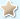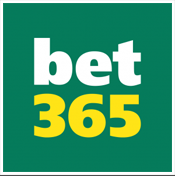# Roulette OddsCasino OffersCasino Bonus Offers

T&Cs Apply, 18+

Latest Casino Articles
Published: 08/04/2012Perhaps more than any other table game, Roulette lends itself quite easily to statistical analysis. There are only 37 possible outcomes (38 in the American version of the game). Each outcome is random and occurs completely independent of all previous results. So far from being rocket science, just a little skill at mathematics is all thats needed to understand Roulette odds.

In the examples that follow, all calculations are based upon the European version of Roulette with a Single Zero and the numbers 1~36 in play. The basic equations presented can be easily adapted for the American version, from series of simple straight-up bets to more complex wagering patterns. Keep in mind, however, that the odds for European Roulette will always be more favourable to the player than those for the American game, owing to a significantly lower House edge.

First, lets define the probability (P) of a winner coming up on a single spin of the wheel as the sum of all numbers bet (B) divided by the total number of possible outcomes (37). This probability can be represented either as a ratio, B:37, or by the equation P = B/37. Using this formula to calculate the odds of one specific number coming up (B=1), the ratio would be 1:37, while the equation would be P = 1/37 = 2.70%. It is no coincidence that the House edge in European Roulette is also 2.70%, because there is one banker slot on the wheel, the Single Zero.

The same method of calculation can be used to find the probability of one of the 18 Black numbers coming up (B=18). The ratio is 18:37 and the equation shows P = 18/37 = 48.65% as the players odds of winning. Since 19 of the numbers are not Black, the House can expect to win 19:37 or P= 19/37 = 51.35% of the time. Again, the difference between the Houses odds and the players (51.35% - 48.65%) is the House edge (2.70%).

The very same calculation applies to all of the other even-money bets at the Roulette table, namely Red, Odd, Even, Low (1~18) and High (19~36). In other words, players should expect to win a little less than half the time when making even-money wagers.

Using this simple formula, it is also easy to calculate that the odds of a winning a wager on a split (pair of numbers) must be 5.4%, a successful street (trio or row) must be 8.1%, and a correctly forecast column of 12 numbers or a sequential dozen must be 32.43%. When expressed as ratios, the respective odds are 2:37, 3:37 and 12:37.

The next step after mastering the basic math for the outcome of a single spin is to learn how to calculate odds for multiple spins. Remember that Roulette is a game of independent events; it has no memory for previous spins, so past results have no influence on future results. How likely is a winner to appear within a specific series of bets?

The formula that answers this question is just a bit more complex: P = 1  {(37-B)/37}N, where N represents the number of spins. Clearly if only one number is selected for one spin, the equation shows that P = 1  {(37-1)/37}1 = 2.70%, which is exactly what should be expected from previous calculations. When betting on Black for one spin, the probability is P = 1  {(37-18)/37}1 = 48.65%, again confirming the previous math.

For a more complex application, consider the popular betting pattern know as the Birthday Strategy, whereby players wager on the day and month of their birth. Someone born on March 15th would bet on 3 and 15, while a person born on October 23rd would bet on 10 and 23. In both of these instances, B=2 and N=1, so doing the calculations using either formula, the odds of winning on a single spin are P = 5.4%.

But what if the Birthday player commits to wagering on the two chosen numbers several times. With each and every spin, the probability of a win is always 5.4%, but the cumulative odds of winning over the course of numerous spins are much higher.

For example, betting on two numbers (B=2) for six spins (N-=6) yields a probability of P = 1  {(37-2)/37}6 = 28.35%. If the series extends to 13 spins (N=13), the probability rises to P = 1  {(37-2)/37}13 = 51.4%, or a little more than half. It is therefore possible to calculate the odds of a Roulette winner on any combination of numbers (B) for any numbers of spins (N) by using the second equation.

Published on: 08/04/2012